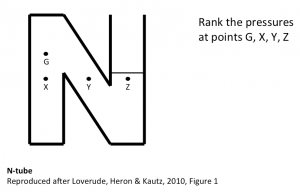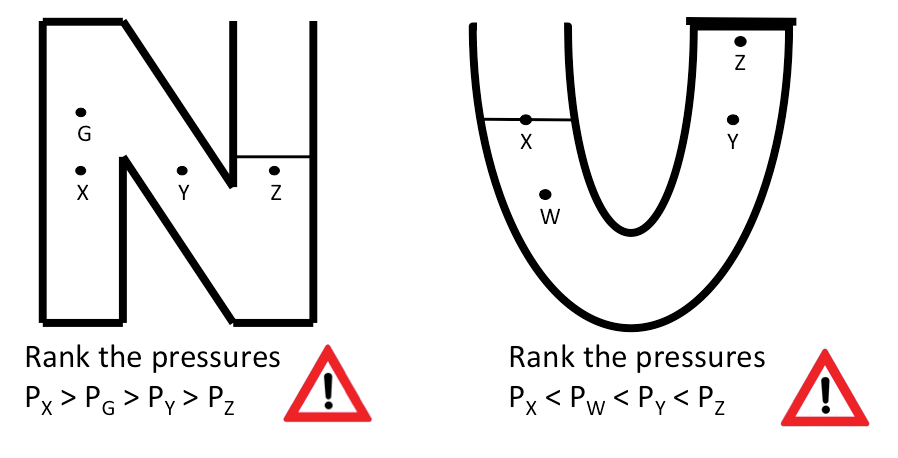# Letter tubes and hydrostatic pressure

How less than 25% of the tested students give consistent answers to these problems.

This is already the third blog post talking about the paper “Identifying and addressing student difficulties with hydrostatic pressure” by Loverude, Heron and Kautz (the first two posts here and here). But I am still a bit in shock by what I read in that paper.

Consider the figure below. A N-shaped tube filled with water.The N-tube problem.

Students are asked to rank the pressure at points G, X, Y, Z.

Because I hate reading electronics papers where they give you the questions and the students’ misconceptions, but don’t tell you what the correct answer would be (how would I know?) I am going to give you the answer, but I’ll assume that you know it anyway. Clearly, points X, Y and Z have the same pressure, whereas the pressure at point G is less.

So what do students say?

A very prominent answer, according to the authors of the study, is that students confuse pressure with weight. Since there is more water above X than above any of the other points, the pressure here seems to have to be highest. And following this logic, the pressure at Z is the smallest (for a sketch of the wrong “h”s that go into this answer, see the figure above).

Using a different-shaped tube, and asked again to rank pressures, students find different results (rather than giving the correct answer, which would be that the pressure at X and Y is the same, the one at W is higher and the one at Z is lower):

Here, many students conclude that the pressure must be increasing from X through W through Y through Z, hence perceiving pressure as varying along the curvature of the letter.

When students in that study were shown both letters together, this is what the typical answers look like:Comparing the N-tube and the U-tube.

The authors find that less than 25% of the students answer these two problems (even when shown side-by-side) consistently. And consistently means just that: They either answer both correctly, OR they answer both of them based on the misconception described for the N-tube, OR they answer both of them based on the misconception described for the U-tube.

This means that 75% of the students in the study didn’t even have a mental model that they consistently used. And those were students who had gone through the standard instruction in hydrostatics. This makes me wonder how this translates to my own students. I have never explicitly talked about these kinds of problems, assuming that students had a full grasp of the material. But clearly this is an assumption that should not be made. But where do we have to start teaching if this is still so fraught with difficulties? Do you have any ideas? Then please let me know.

## 3 thoughts on “Letter tubes and hydrostatic pressure”

1. Pingback: Water seeks its level |